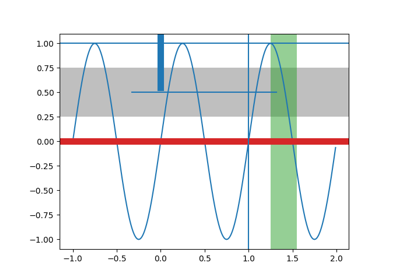# matplotlib.pyplot.axvspan¶

matplotlib.pyplot.axvspan(xmin, xmax, ymin=0, ymax=1, \*\*kwargs)[source]

Add a vertical span (rectangle) across the axes.

Draw a vertical span (rectangle) from xmin to xmax. With the default values of ymin = 0 and ymax = 1. This always spans the yrange, regardless of the ylim settings, even if you change them, e.g., with the set_ylim() command. That is, the vertical extent is in axes coords: 0=bottom, 0.5=middle, 1.0=top but the x location is in data coordinates.

Parameters: xminscalarNumber indicating the first X-axis coordinate of the vertical span rectangle in data units. xmaxscalarNumber indicating the second X-axis coordinate of the vertical span rectangle in data units. yminscalar, optionalNumber indicating the first Y-axis coordinate of the vertical span rectangle in relative Y-axis units (0-1). Default to 0. ymaxscalar, optionalNumber indicating the second Y-axis coordinate of the vertical span rectangle in relative Y-axis units (0-1). Default to 1. rectanglePolygonVertical span (rectangle) from (xmin, ymin) to (xmax, ymax). **kwargsOptional parameters are properties of the class Polygon.

axhspan
Add a horizontal span across the axes.

Examples

Draw a vertical, green, translucent rectangle from x = 1.25 to x = 1.55 that spans the yrange of the axes.

>>> axvspan(1.25, 1.55, facecolor='g', alpha=0.5)


## Examples using matplotlib.pyplot.axvspan¶